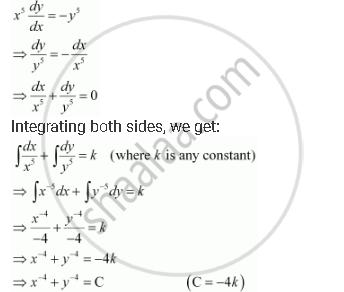HSC Science (Computer Science) 12th Board ExamMaharashtra State Board
Share

# For the Differential Equations Find the General Solution: X^5 Dy/Dx = - Y^5 - HSC Science (Computer Science) 12th Board Exam - Mathematics and Statistics

ConceptMethods of Solving First Order, First Degree Differential Equations Differential Equations with Variables Separable

#### Question

For the differential equations find the general solution:

x^5 dy/dx = - y^5

#### Solution

The given differential equation is:Is there an error in this question or solution?

#### APPEARS IN

NCERT Solution for Mathematics Textbook for Class 12 (2018 to Current)
Chapter 9: Differential Equations
Q: 8 | Page no. 396
Solution For the Differential Equations Find the General Solution: X^5 Dy/Dx = - Y^5 Concept: Methods of Solving First Order, First Degree Differential Equations - Differential Equations with Variables Separable.
S